【判断题】阿利·伯克级驱逐舰是航母编队的护卫兵力，配备宙斯盾作战系统，是世界上最先进的驱逐舰之一。宙斯盾作战系统，主要以AN/SPY-1D被动相控阵雷达，结合MK-41型垂直发射系统，承担着编队防空、反导等使命任务。
A.

B.（）是基于角动量守恒的理论而设计的一种用来传感与维持方向的装置。
A.

B.

C.

D.

A.

B.

C.

D.

A.

B.

A.

B.

C.

D.

()可实现运行期间胶带姿态调节，防止跑偏，具有自动张紧胶带功能，回采期间自移，避免工作面产量受转载机搭接长度制约。
A.

B.

C.

D.

A.

B.

A.

B.

C.

D.

A.

B.

C.

D.

A.
SOP
B.
QFP
C.
DIP
D.
SOT

A.

B.

C.

D.

A.

B.

C.

D.

A.

B.

（）一般提供润滑油的油温、油位、油压等状态参量的监测，是判断泵站运行状态健康性的重要依据。
A.

B.

C.

D.

（）是由于离心力的作用引起皮带偏离了胶带输送机的中线，从而导致偏向一侧的现象。
A.

B.

C.

D.

A.

B.

C.

D.

A.

B.

DIP16封装集成电路，文字面朝向自己，引脚朝外，缺口放左边，则左下方为（ ）脚，逆时针方向识读其他引脚。
A.
16
B.
1
C.
9
D.
8
______是位于主体之后的，离观众更远的展现故事发生场景氛围的景物。是环境的重要组成部分。
A.

B.

C.

D.

（）技术是以煤层地质构造数字模型作为导航地图，确定采煤机及其截割滚筒与工作面煤层顶底板的位置关系，实现采煤机动态定位。
A.

B.

C.

D.

A.

B.

C.

D.

（）是指煤块里混合金属、石头等杂质，在运输过程中造成堵塞，尤其是机头机尾和转载口处，导致物料堆积的一种故障现象。
A.

B.

C.

D.

（）包括水质、乳化油油位、乳化液液位、乳化液浓度等方面的监测，以稳定乳化液的配比浓度。
A.

B.

C.

D.

A.

B.

C.

D.

A.

B.

C.

D.

A.

B.

C.

D.

A.

B.

C.

D.

A.

B.

C.

D.

A.

B.

BGA封装，即球栅阵列封装，其引脚形状为（ ）。
A.

B.

C.
J型
D.

A.

B.

（）一般通过过滤系统进液和出液压力差值的监测，来判断滤芯纳污量，为自动反冲洗和更换滤芯提供提示信息。
A.

B.

C.

D.

A.

B.

A.

B.

DIP14封装集成电路，一般第（ ）脚接电源正极。
A.
1
B.
8
C.
14
D.
7

A.

B.

C.

D.

A.

B.

A.

B.

C.

D.

A.

B.

（）在线监测诊断，一般是通过传感器，将振动或噪声等表征机械状态的特征参量转化为电信号，经过放大采集、信号处理和分析后，对故障信息或故障零部件进行报警或诊断。
A.

B.

C.

D.

A.

B.

C.

D.

A.

B.

SOP封装、QFP封装都是（ ）形状的引脚。
A.

B.
J型
C.

D.

A.

B.

A.

B.

A.

B.

C.

D.

（ ）为多芯片组件封装。
A.
QFP
B.
MCM
C.
CSP
D.
SOP

A.

B.

A.

B.

C.

D.

A.

B.

A.

B.

A.

B.

A.

B.

A.
51KΩ
B.
56KΩ
C.
560Ω
D.
5.6KΩ

A.

B.

C.

D.

A.

B.

A.

B.

A.

B.

PCBA生产过程中使用的治具是过炉治具。
A.

B.

A.

B.

A.

B.

A.

B.

C.

D.

A.

B.

A.

B.

C.

D.

A.

B.

QC是品质控制的意思，包括IQC、IPQC、FQC和 。

A.

B.

A.

B.

A.

B.

QE即 工程师。

A.

B.

A.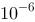B.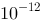C.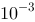D.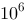AOI自动光学检测仪，是基于光学原理，对焊接生产中的常见缺陷进行检测的设备。
A.

B.

A.

B.

A.

B.

A.B.C.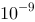D.A.
+3%
B.
－3‰
C.
－3%
D.
+3‰

A.

B.

A.

B.

A.

B.

C.

D.

A.

B.

C.

D.

A.

B.

C.

D.

A.

B.

A.

B.

C.

D.

A.

B.

C.

D.

A.

B.

C.

D.

A.

B.

C.

D.

A.
JZG
B.
DBG
C.
YBG
D.
YLHZ

A.

B.

C.

D.

A.

B.

C.

D.

A.

B.

C.

D.打开"刷刷题APP"，使用更方便